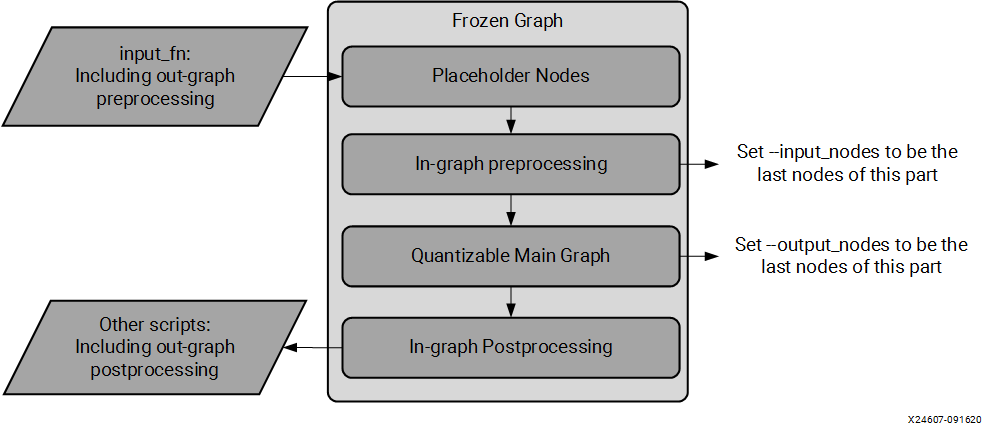# Quantizing the Model Using vai_q_tensorflow - 2.0 English

## Vitis AI User Guide (UG1414)

Document ID
UG1414
Release Date
2022-01-20
Version
2.0 English

Run the following commands to quantize the model:

``````\$vai_q_tensorflow quantize \
--input_frozen_graph  frozen_graph.pb \
--input_nodes    \${input_nodes} \
--input_shapes   \${input_shapes} \
--output_nodes   \${output_nodes} \
--input_fn  input_fn \
[options]
``````

The input_nodes and output_nodes arguments are the name list of input nodes of the quantize graph. They are the start and end points of quantization. The main graph between them is quantized if it is quantizable, as shown in the following figure.

Figure 1. Quantization Flow for TensorFlowIt is recommended to set `–input_nodes` to be the last nodes of the preprocessing part and to set `-output_nodes` to be the last nodes of the main graph part because some operations in the pre- and postprocessing parts are not quantizable and might cause errors when compiled by the Vitis AI quantizer if you need to deploy the quantized model to the DPU.

The input nodes might not be the same as the placeholder nodes of the graph. If no in-graph preprocessing part is present in the frozen graph, the placeholder nodes should be set to input nodes.

The `input_fn` should be consistent with the placeholder nodes.

[options] stands for optional parameters. The most commonly used options are as follows:

weight_bit
Bit width for quantized weight and bias (default is 8).
activation_bit
Bit width for quantized activation (default is 8)
method
Quantization methods, including 0 for non-overflow, 1 for min-diffs, and 2 for min-diffs with normalization. The non-overflow method ensures that no values are saturated during quantization. The results can be affected by outliers. The min-diffs method allows saturation for quantization to achieve a lower quantization difference. It is more robust to outliers and usually results in a narrower range than the non-overflow method.